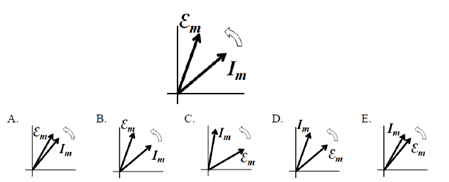Problem: The phasor diagram shown here is for a driven, series RLC circuit when the driving frequency is three times the circuit's resonant frequency.Which of the following would be the phasor diagram for the same RLC circuit if the driving frequency is one third of the resonant frequency?

FREE Expert Solution
79% (258 ratings)
Problem Details

The phasor diagram shown here is for a driven, series RLC circuit when the driving frequency is three times the circuit's resonant frequency.Which of the following would be the phasor diagram for the same RLC circuit if the driving frequency is one third of the resonant frequency?